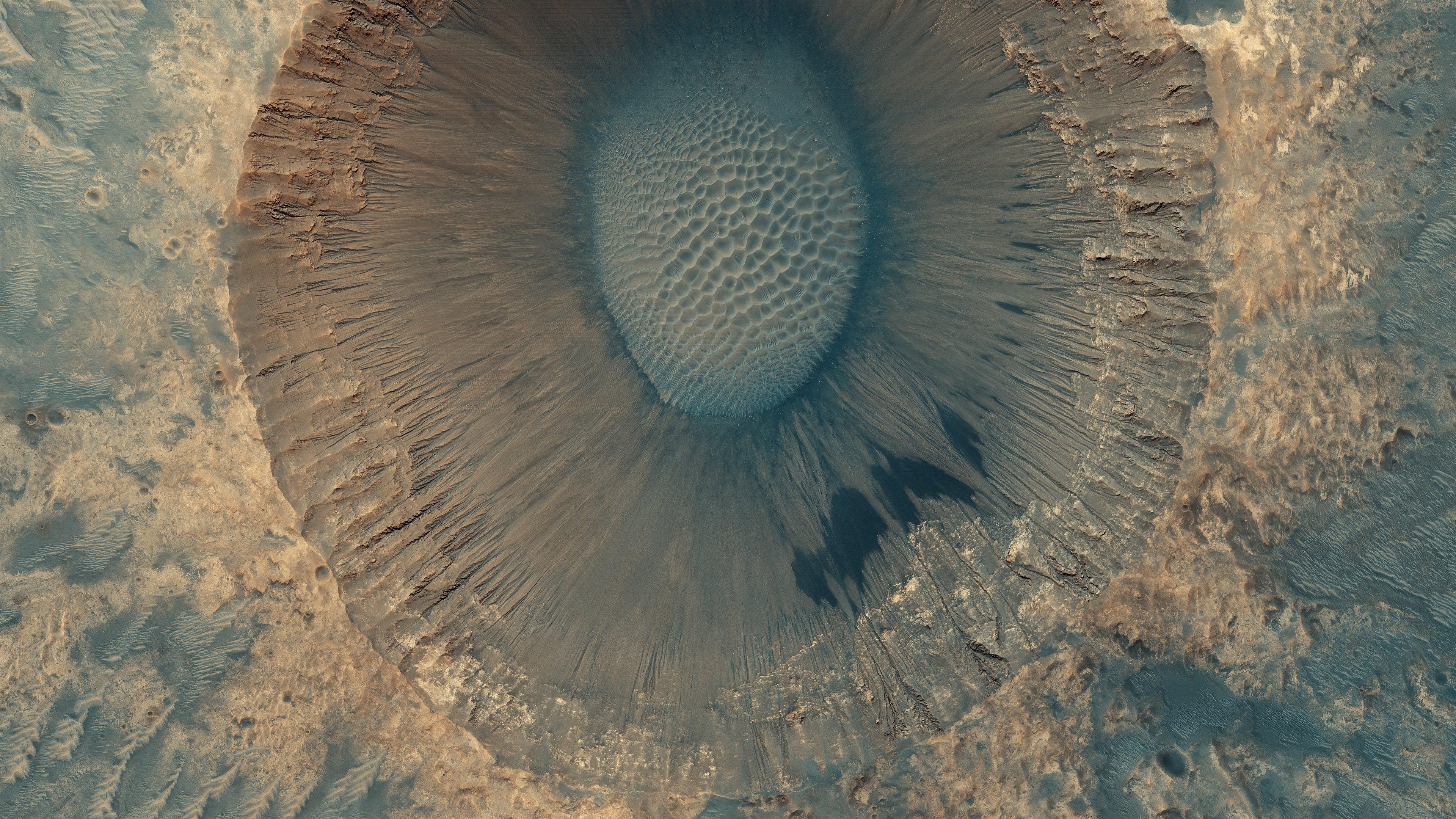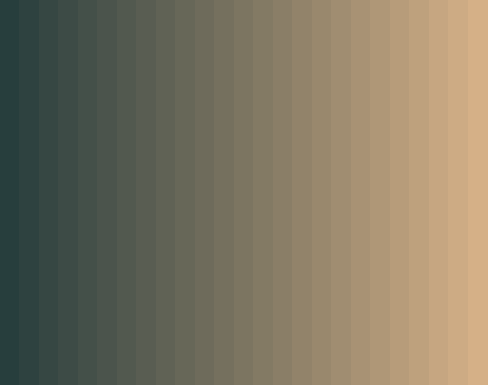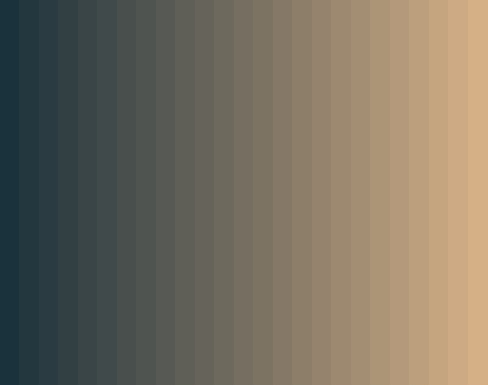## Mars landscape color palette

I came across this gorgeous image of Mars landscape and I was compelled to create a color palette. This image was captured by HiRISE (NASA) and processed by Seán Doran. I created this color scale with support from Chroma.js Color Palette Helper.``````library(ggplot2)
library(hexbin)
library(cowplot)
library(chroma)

# color palettes
chroma::show_col(interp_colors(25, colors=c("#3a5251",  "#d5b087"), interp="bezier"))
chroma::show_col(interp_colors(25, colors=c("#19323b", "#d5b087"), interp="bezier"))
````````````# plot
green_ish <- ggplot(data.frame(x = rnorm(100000), y = rnorm(100000)), aes(x = x, y = y)) +
geom_hex() + coord_fixed() + theme_void() +
low = "#273e3d",
high = "#d5b087")

blue_ish <- ggplot(data.frame(x = rnorm(100000), y = rnorm(100000)), aes(x = x, y = y)) +
geom_hex() + coord_fixed() + theme_void() +